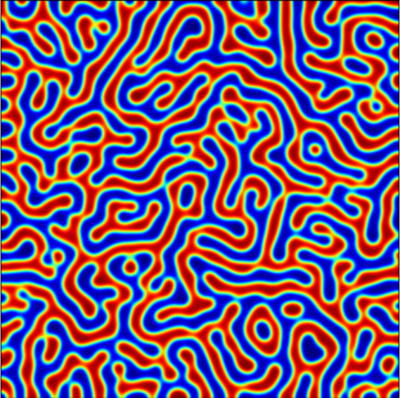Thomas Wanner
Department of Mathematical Sciences
George Mason University
4400 University Drive, MS 3F2
Fairfax, Virginia 22030, USA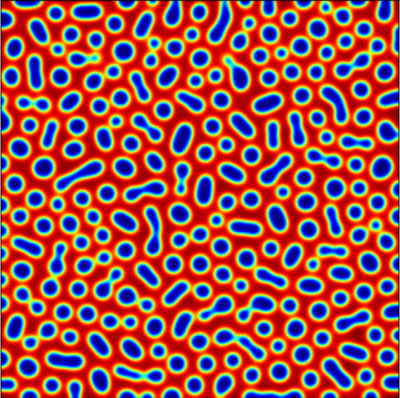## Nucleation in a Ternary Cahn-Morral Model

Part of my research has been focused on mathematical models for phase separation in multi-component alloys, in particular on the phenomenon of nucleation. Below you can see some movies of simulated phase separation in a ternary Cahn-Morral model in two space dimensions and with components $u$, $v$, and $w$, for a variety of values of the total mass $\mu_u$. The remaining two components have masses $\mu_v = 0.2 - \mu_u$ and $\mu_w = 0.8$. To see the movies, just click on the images.

$\mu_u = 0.100$ $\mu_u = 0.105$ $\mu_u = 0.110$$\mu_u = 0.115$ $\mu_u = 0.120$ $\mu_u = 0.125$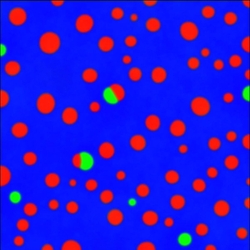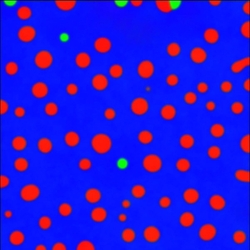$\mu_u = 0.130$ $\mu_u = 0.135$ $\mu_u = 0.140$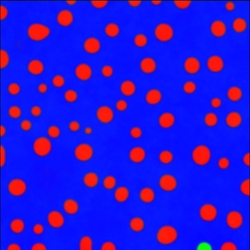The following simulations are for the same mass values, but for larger interaction length. They show the formation of the nuclei more clearly.

$\mu_u = 0.100$ $\mu_u = 0.105$ $\mu_u = 0.110$$\mu_u = 0.115$ $\mu_u = 0.120$ $\mu_u = 0.125$$\mu_u = 0.130$ $\mu_u = 0.135$ $\mu_u = 0.140$